# NCRT Miscellaneous Excercise

Question 1: The relation f is defined by f(x)=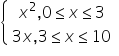The relation g is defined by g(x)=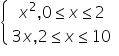Show that f is a function and g is not a function.

Answer 1: The relation f is defined as

f(x)=For

0 ≤ x < 3, f(x) = x2

3 < x ≤ 10, f(x) = 3x

Also, at x = 3, f(x) = 32 = 9

or f(x) = 3 × 3 = 9

i.e., at x = 3, f(x) = 9

Therefore, for 0 ≤ x ≤ 10,  images of f(x) are unique. Thus, the given relation is a function.

The relation g is defined as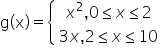It can be observed that for x = 2, g(x) = 22 = 4 and g(x) = 3 × 2 = 6

Question 2: If f(x) = x2, find.       f(1.1)-f(1)/(1.1-1)

Therefore f(1.1)-f(1)/(1.1-1)

=(1.1)2-(1)2/(1.1-1)

=1.21-1/0.1

=0.21/0.1=2.1

Question 3: Find the domain of the function      f(x)=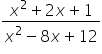Answer 3: The given function is f(x)=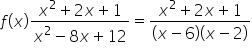As we can see Function f is defined for all real numbers except at x = 6 and x = 2. Hence, the domain of f is R – {2, 6}.

Question 4: Find the domain and the range of the real function f defined by (x) =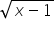Answer 4: The given real function is (x) =As can be seen thatis defined for x ≥ 1.

Therefore, the domain of f is the set of all real numbers ≥ to 1 i.e., the domain of f = [1, ∞).

As x ≥ 1 ⇒ (x – 1) ≥ 0 ⇒≥ 0

Therefore, the range of f is the set of all real numbers ≥ 0 i.e., the range of f = [0, ∞).

Question 5: Find the domain and the range of the real function f defined by f (x) = |x – 1|.

Answer 5:  f (x) = |x – 1|.

It is clear that |x – 1| is defined for all real numbers.

∴ Domain of f = R

Also, for x ∈ R, f(x) assumes all real numbers.

Hence, the range of f is the set of all non-negative real numbers.

Question 6:

Let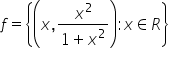be a function from R into R. Determine the range of f.

Answer 6: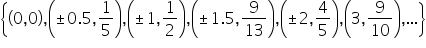The range of f is the set of all second elements. It can be observed that all these elements are ≥ 0 but less than 1.

Thus, range of f = [0, 1)

Question 7:

Let f, g: R → R be defined, respectively by

f(x) = x + 1,

g(x) = 2x – 3.

Find f + g, f – g and f/ g.

Answer 7: f, g: R → R is defined as f(x) = x + 1, g(x) = 2x – 3

(f + g) (x) = f(x) + g(x) = (x + 1) + (2x – 3) = 3x – 2

∴ (f + g) (x) = 3x – 2

(f – g) (x) = f(x) – g(x) = (x + 1) – (2x – 3) = x + 1 – 2x + 3 = – x + 4

∴ (f – g) (x) = –x + 4

(f/g)(x)=f(x)/g(x), g(x) ≠0, x∈ R

∴ (f/g)(x)=x+1/2x-3 ≠ 0   or  2x≠3

∴ (f/g)(x)=x+1/2x-3, x≠3/2

Question 8:

Let f = {(1, 1), (2, 3), (0, –1), (–1, –3)} be a function from Z to Z defined by f(x) = ax + b, for some integers a, b. Determine a, b.

f = {(1, 1), (2, 3), (0, –1), (–1, –3)} and f(x) = ax + b

For ist element of set i.e.  (1, 1) ∈ f ⇒ f(1) = 1 ⇒ a × 1 + b = 1

⇒ a + b = 1

(0, –1) ∈ f ⇒ f(0) = –1 ⇒ a × 0 + b = –1

⇒ b = –1

On substituting b = –1 in a + b = 1,

We obtain a + (–1) = 1 ⇒ a = 1 + 1 = 2. Thus, the respective values of a and b are 2 and –1.

Question 9: Let R be a relation from N to N defined by R = {(a, b): a, b ∈ N and a = b2}. Are the following true?

1. (a, a) ∈ R, for all a ∈ N
2.  (a, b) ∈ R, implies (b, a) ∈ R
3.  (a, b) ∈ R, (b, c) ∈ R implies (a, c) ∈ R.

R = {(a, b): a, b ∈ N and a = b2}

1. We can see that that 2 ∈ N; however, 2 ≠ 22 = 4.

Therefore, the statement “(a, a) ∈ R, for all a ∈ N” is not true.

1. We can see  that (9, 3) ∈ N because 9, 3 ∈ N and 9 = 32. Now, 3 ≠ 92 = 81; therefore, (3, 9) ∉ N

Therefore, the statement “(a, b) ∈ R, implies (b, a) ∈ R” is not true.

1.  As can be seen that (9, 3) ∈ R, (16, 4) ∈ R because 9, 3, 16, 4 ∈ N and 9 = 32 and 16 = 42.

Now, 9 ≠ 42 = 16; therefore, (9, 4) ∉ N

Therefore, the statement “(a, b) ∈ R, (b, c) ∈ R implies (a, c) ∈ R” is not true.

Question 10: Let A = {1, 2, 3, 4}, B = {1, 5, 9, 11, 15, 16} and f = {(1, 5), (2, 9), (3, 1), (4, 5), (2, 11)}. Are the following true?

1. f is a relation from A to B
2.  f is a function from A to B.

Answer 10: A = {1, 2, 3, 4} and B = {1, 5, 9, 11, 15, 16}

∴ A × B = {(1, 1), (1, 5), (1, 9), (1, 11), (1, 15), (1, 16), (2, 1), (2, 5), (2, 9), (2, 11),(2, 15), (2, 16), (3, 1), (3, 5), (3, 9), (3, 11), (3, 15), (3, 16), (4, 1), (4, 5), (4, 9), (4, 11), (4, 15), (4, 16)}

It is given that f = {(1, 5), (2, 9), (3, 1), (4, 5), (2, 11)}

1. A relation from a non-empty set A to a non-empty set B is a subset of the Cartesian product A × B.

It is observed that f is a subset of A × B.

Thus, f is a relation from A to B.

2. Since the same first element i.e., 2 corresponds to two different images i.e., 9 and 11, relation f is not a function.

Question 11:Let f be the subset of Z × Z defined by f = {(ab, a + b): a, b ∈ Z}. Is f a function from Z to Z: justify your answer.

Answer 11: The relation f is defined as f = {(ab, a + b): a, b ∈ Z}

We know that a relation f from a set A to a set B is said to be a function if every element of set A has unique images in set B.

Since 2, 6, –2, –6 ∈ Z, (2 × 6, 2 + 6), (–2 × –6, –2 + (–6)) ∈ f i.e., (12, 8), (12, –8) ∈ f

It can be seen that the same first element i.e., 12 corresponds to two different images i.e., 8 and –8. Thus, relation f is not a function.

Question 12: Let A = {9, 10, 11, 12, 13} and let f: A → N be defined by f(n) = the highest prime factor of n. Find the range of  f.

Answer 12: A = {9, 10, 11, 12, 13} f: A → N is defined as f(n) = The highest prime factor of n

Prime factor of 9 = 3

Prime factors of 10 = 2, 5

Prime factor of 11 = 11

Prime factors of 12 = 2, 3

Prime factor of 13 = 13

∴ f(9) = The highest prime factor of 9 = 3

f(10) = The highest prime factor of 10 = 5

f(11) = The highest prime factor of 11 = 11

f(12) = The highest prime factor of 12 = 3

f(13) = The highest prime factor of 13 = 13

The range of f is the set of all f(n), where n ∈ A.

∴ Range of f = {3, 5, 11, 13}

Related Keywords